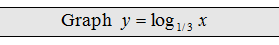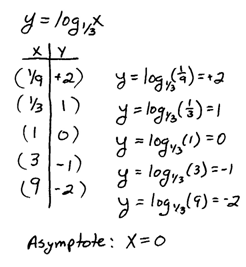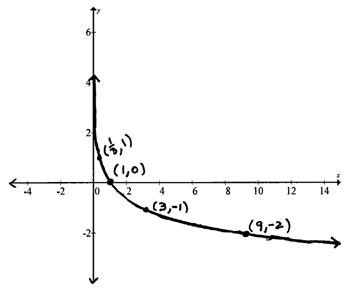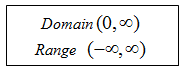## Algebra

Showing posts with label natural. Show all posts
Showing posts with label natural. Show all posts

### The Natural Exponential Function

In this section we will introduce the natural base and define and graph the natural exponential function.
YouTube playlist for steps covering exponential functions and their graphsThis number is so special, just as is pi, that it has its own button on your scientific calculator. Try finding the e^x button on your calculator and using it to calculate e.

Evaluate.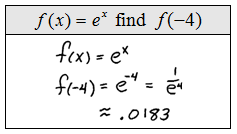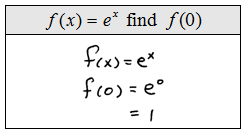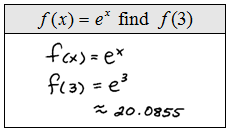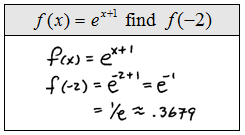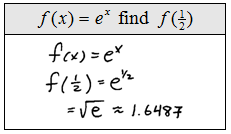Graph the following exponential functions. State the domain, range, and label the horizontal asymptote.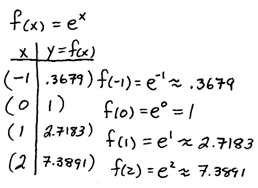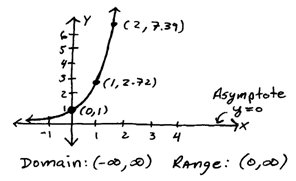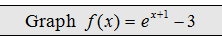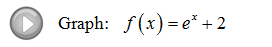### Graphing Logarithmic Functions

One way to graph logarithmic functions is to first graph its inverse exponential. Then use your knowledge about the symmetry of inverses to graph the logarithm.

YouTube Playlist: Logarithms and their Graphs

Recall that inverses are symmetric about the line y = x. For example,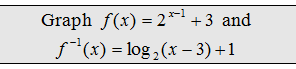Step 1: Find some points on the exponential f(x). The more points we plot the better the graph will look.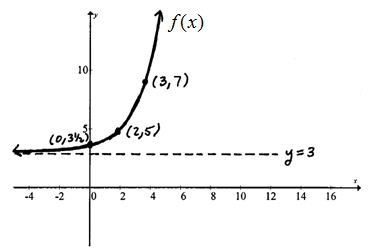Step 2: Switch the x and y values to obtain points on the inverse.Step 3: Determine the asymptote.In practice, we use a combination of techniques to graph logarithms.  We can use our knowledge of transformations, techniques for finding intercepts, and symmetry to find as many points as we can to make these graphs. General guidelines follow:

1. Graph the vertical asymptote. All logarithmic functions of the formhave a vertical asymptote at x = h.

2. Find the x- and y-intercepts if they exist. To find x-intercepts set y = f(x) to zero and to find y-intercepts set x = 0.

3. Plot a few more points and graph it.

Graph the following logarithmic functions. State the domain and range.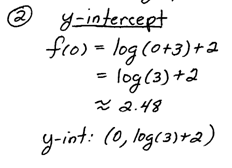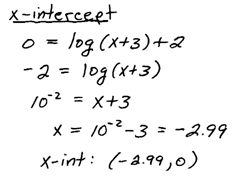In the previous solved problem, make a note of the rigid transformations.  If we start with the basic graph y = log(x) then the first translation is a shift to the left 3 units y = log(x+3).  Next we see a vertical shift up 2 units y = log(x+3)+2 .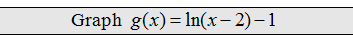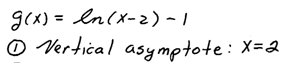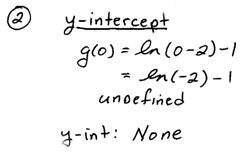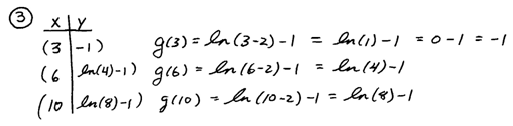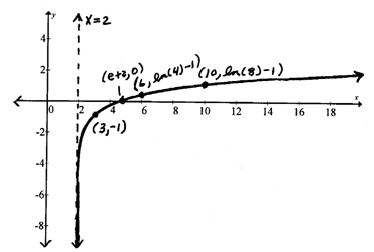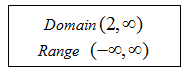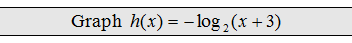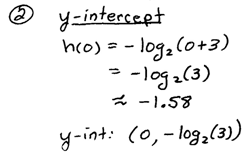In the above problem there was a reflection about the x-axis as well as a shift to the left 3 units.